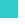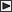BRI Research Paper

No.80

Studies on Probabilistic Spread of Fire.

Y.Aoki; November, 1978. 52p.

Abstract

In the present paper an attempt is made to accurately understand the spread and progress of fire by dividing the spread of a fire within a single building into several phases and by relating them to one another from the point of view of theory of probability.

 In chapter 1, a more adequate form of fire spread model for application by collecting and classifying data of actual housing fires is composed as a stochastic state-transition system. The system of differential equations which describes the behaviour of this stochastic state-transition corresponding fire spread is derived and its solution is obtained. Similarly the equation of survival probability for each phase at each time is solved. In chapter 2, as a simplified measure of the above stochastic fire spread system, the average arrival time and the average extinguishment time for each phase are obtained from the above solution. In chapter 3, the unknown parameters in our model are estimated with data consisted of a total of 335 fire-cases randomly sampled from actual fires. And the behaviour of the value of survival probability for each phase is obtained. In chapter 4, the instantaneous extinguishment rate and instantaneous transition rate for each phase are estimated with the result of the previous chapter. The behaviour of existence probability for each phase is obtained with the above estimated parameters, and the differences between the properties of fire-spread process in Phases 1 through 3 and one of the process in Phase 4 and subsequent phases are clarified. In chapter 5, the differences in fire-spread process among the building types of structure are shown by estimating parameters for each type of structure and it is clear that when the trend of fire-extend up to a flashover is viewed probabilistically, a fire extends most quickly in a building of wood-en construction and a fire in a building of fireproof construction extends most slowly. In chapter 6, a model for fire escape is formulated and an equation of relation between the average escape time and a parameter in this model is obtained. With those result, the escape-failure probability which is one of measures of danger to human life is obtained. A comparison of the escape-failure probabilities for each type of structure with the result in previous chapter reveals that wooden construction is more dangerous to human life than fireproof construction. In chapter 7, a model for fire-spread accompanied by losses is formulated.And the economic loss is analyzed in a model case of house and it is shown that the amount of economic loss increases remarkably in the range of 5 minutes to 20 minutes after ignition and its trend also indicates the importance of the fire-fighting in the early stages from a view point of probabilistic approach.LinksSitemapInquiryJapanese HP

BUILDING RESEARCH INSTITUTE, JAPAN